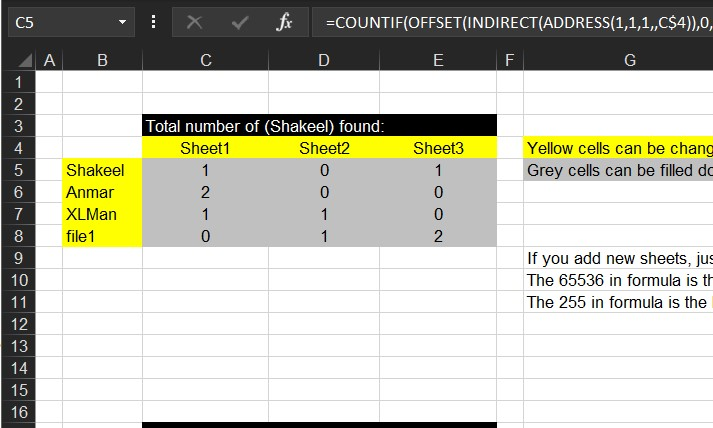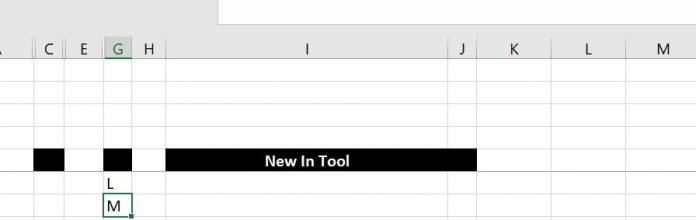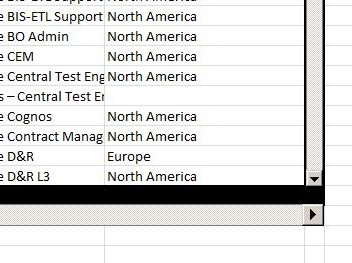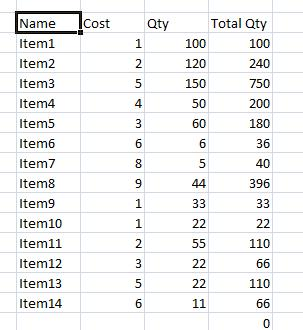## Search across sheets in functionJust when you need to search for a thing across sheets in a workbook. I am bored, so I am adding articles from my old archive.

## Get column name (columnname as A,B,C, etc) as input inside cellExcel already has the formula ROW() =Row() Which as we may already know will give us the number of the row we are in, if you add no parameters However, …

## iframe in Excel (XLiFrame)This is the iframe in Excel, if you are familiar with the HTML concept of iframe, you will understand this one right away, it is basically the same project as …

## Rotate table using 1 formulaRotating table (Transpose) using functions so that the table is updated once the source table is updated. Also can be used as automatically rotating tables with variable number of rows …